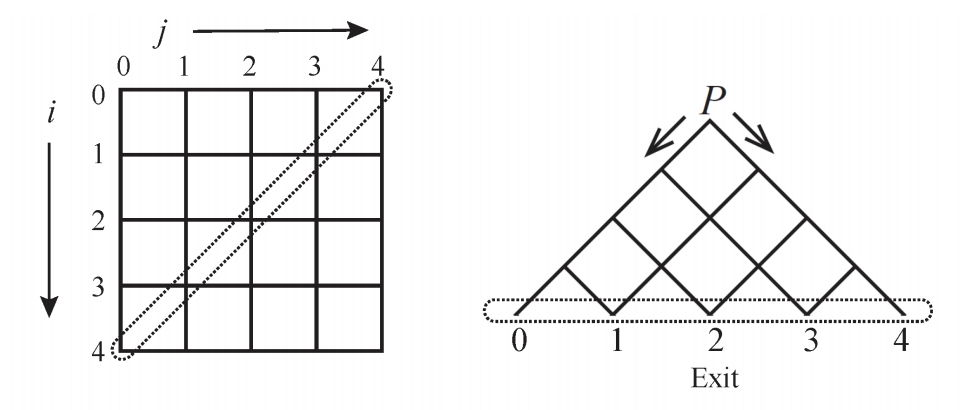시간 제한 메모리 제한 제출 정답 맞은 사람 정답 비율
3 초 1024 MB 2 2 2 100.000%

## 문제

Consider an n×n grid where nodes are labelled as (i, j) for 0 ≤ i, j < n. We rotate it 45 degree in clockwise direction and keep only its top half part. Then you get a pyramid, as shown in Figure 1. All of the nodes laid on the anti-diagonal of the grid have labels (n − 1 − j, j) for 0 ≤ j < n. They are located at the bottom line of the pyramid. For simplicity and clarity, we name node (n − 1 − j, j) as exit j. Node (0, 0) is called the starting point (labelled as P in Figure 1). When you insert a ball from the starting point, this ball will roll down to some of the exits. A ball located at node (i, j) can only roll down to either node (i + 1, j) or node (i, j + 1), and the ball shall never be broken or split. There is a tiny switch equipped on every node other than the exits that controls the move direction of the ball, and this switch always works well. The switch has exactly two states: either L or R, indicates that the ball can move to node (i + 1, j) or (i, j + 1), respectively. After the ball leaves this node, the switch changes immediately to the other state. The default setting for a switch is at L.Figure 1: An example for n = 5.

When you insert the first ball into P, this ball will reach exit 0, and the states of nodes (i, 0) for 0 ≤ i < n − 1 are now all R’s. Then you insert the second, third, and so on so forth, one by one, until the kth ball finishes. The status of every switch accumulates with these balls. Please write a program to determine the number of the exit point for the kth ball.

## 입력

The first line of the input is a number that specifies the number of test cases. Each test case has only one line that gives you two space-delimited numbers n and k.

## 출력

Please output the exit number of the kth ball in one line.

## 제한

• There are at most 20 test cases.
• 1 ≤ n ≤ 104.
• 1 ≤ k ≤ 108.

## 예제 입력 1

2
5 1
5 2


## 예제 출력 1

0
1


## 예제 입력 2

3
5 3
5 4
5 5


## 예제 출력 2

2
3
2